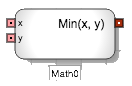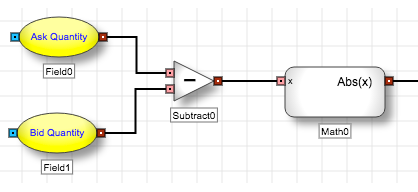# Arithmetic blocks

Arithmetic blocks

# Math blockThe Math block provides the user methods for trigonometric, logarithmic, and other common mathematical functions.

When the user selects a function from the drop-down menu the Math block exposes the corresponding number of input ports and output ports. For example, if the user selects Log (x, base), then the Math block exposes two input ports and one output port.

Example The Math block configure to use the Abs(x) function outputs the absolute value of the input received from the Subtract block, ensuring that downstream logic can rely on a positive value for the difference between the bid and ask quantities.## Math functions

Function Description
Abs(x) Returns the absolute value of the specified number.
Acos(x) Returns the angle whose cosine is the specified number.
Asin(x) Returns the angle whose sine is the specified number.
Atan(x) Returns the angle whose tangent is the specified number.
Atan2(y,x) Returns the angle whose tangent is the quotient of two specified numbers.
BigMul(x,y) Produces the full product of two numbers.
Ceiling(x) Returns the smallest integral value that is greater than or equal to the specified decimal number.
Cos(x) Returns the cosine of the specified angle.
Cosh(x) Returns the hyperbolic cosine of the specified angle.
DivRem(x,y,rem) Calculates the quotient of two integers and also returns the remainder in an output parameter.
E Represents the natural logarithmic base, specified by the constant, e.
Exp(x) Returns e raised to the specified power.
Floor(x) Returns the largest integer less than or equal to the specified decimal number.
IEEERemainder(x,y) Returns the remainder resulting from the division of a specified number by another specified number.
Log(x,base) Returns the logarithm of a specified number in a specified base.
Log(x)

Returns the natural (base e) logarithm of a specified number.

Log10(x) Returns the base 10 logarithm of a specified number.
Max(x,y) Returns the larger of two integers.
Min(x,y) Returns the smaller of two integers.
PI Represents the ratio of the circumference of a circle to its diameter, specified by the constant, π.
Pow(x,y) Returns a specified number raised to the specified power.
Sign(x) Returns a value indicating the sign of a decimal number.
Sin(x) Returns the sine of the specified angle.
Sinh(x) Returns the hyperbolic sine of the specified angle.
Sqrt(x) Returns the square root of a specified number.
Tan(x) Returns the tangent of the specified angle.
Truncate(x) Calculates the integral part of a specified decimal number.

## Block properties

To customize the block, update the Block Properties.

Property Description
Name Name to display beneath the block on the ADL canvas
MathFunction Mathematical function for the block's calculation